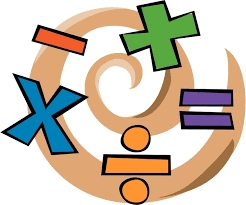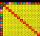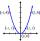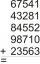# Expressions with variable

This is algebra. Let n represent an unknown number and write the following expressions:

1. 4 times the sum of 7 and the number x
2. 4 times 7 plus the number x
3. 7 less than the product of 4 and the number x
4. 7 times the quantity 4 more than the number x
5. 4 times the quantity 7 less than the number x

Result

a = (Correct answer is: 4(7+x))b = (Correct answer is: 4*7+x)c = (Correct answer is: 4*x-7)d = (Correct answer is: 7(4+x))e = (Correct answer is: 4(x-7))#### Solution:

$a=4(7+x)$
$b=4 \cdot \ 7+x$
$c=4 \cdot \ x-7$
$d=7(4+x)$
$e=4(x-7)$We would be pleased if you find an error in the word problem, spelling mistakes, or inaccuracies and send it to us. Thank you!Tips to related online calculators
Need help calculate sum, simplify or multiply fractions? Try our fraction calculator.

## Next similar math problems:

• ExpressionsLet k represent an unknown number, express the following expressions: 1. The sum of the number n and two 2. The quotient of the number n and nine 3. Twice the number n 4. The difference between nine and the number n 5. Nine less than the number n
• Algebra problemThis is algebra. Let n represent an unknown number. 1. Eight more than the number n 2. Three times the number n 3. The product of the number n and eight 4. Three less than the number n 5. Three decreased by the number n
• Unknown numberIf I reduced the sum of the numbers 70 and the unknown number three times, I would get 100. what is the unknown number?
• ProductThe sum and the product of three integers is 6. Write largest of them.
• Unknown numberSamuel wrote unknown number. Then he had add 200000 to the number and the result multiply by three. When it calculated he was surprised, because the result would have received anyway, if write digit to the end of original number. Find unknown number.
• Let xLet x represent one quantity. State what that quantity represents. Express the second quantity in terms of x. The length of the rectangle is 4 inches less than 8 times the width.
• Unknown xIf we add to unknown number 21, then divide by 6 and then subtract 51, we get back an unknown number. What is this unknown number?
• Unknown number 7Calculate unknown number whose 12th power when divided by the 9th power get a number 27 times greater than the unknown number. Determine the unknown number.Fill letters instead of digits so the indicated sum (equal letters represent equal digits). What number is hidden under the letter J? A A H A H O A H O J -------------------------- 4 3 2 1
• Write 3Write a real world problem involving the multiplication of a fraction and a whole number with a product that is between 8 and 10 then solve the problem
• Unknown number 716% of the unknown number is by 21 less than unknown number itself. Determine the natural unknown number.
• Unknown numberDetermine the unknown number that is equal to a quarter of the fifth of number, which is by 152 more than unknown number.
• Prime divisorsFind 2/3 of the ratio of the sum and the product of all prime divisors of the number 120.
• Product of the sum and differenceCalculate the product of the sum and difference of numbers -7 and -2.
• One-thirdA one-third of unknown number is equal to five times as great as the difference of the same unknown number and number 28. Determine the unknown number.
• Unknown number 5I think unknown number. If we enlarge it five times then subtract 3 and result decreases by 75% we get the number by one greater than the number I think. What number am I thinking?
• FractionsThree-quarters of an unknown number are 4/5. What is 5/6 of this unknown number?